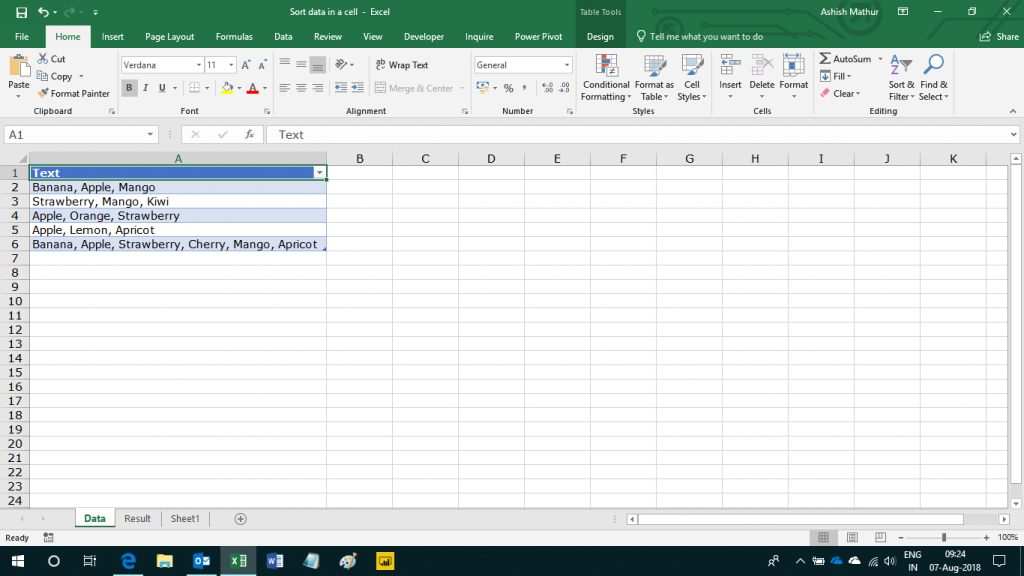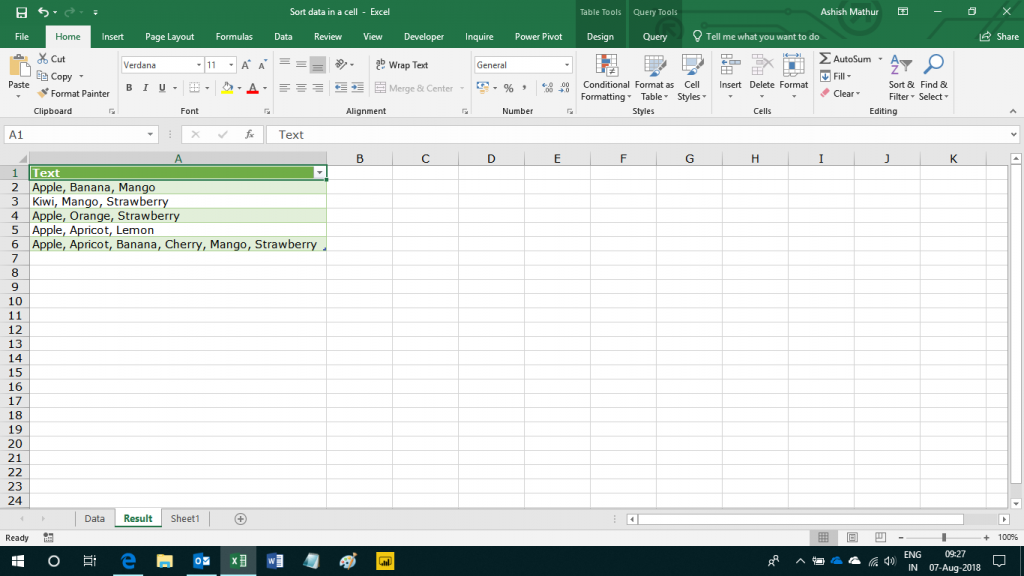Assume a single column dataset as shown below.  As you can observe, there are multiple entries appearing the same cell separated by commas.The objective is to sort, in ascending order, the entries in each cell.  The expected result is shown below.I have solved this problem using Power Query a.k.a Data > Get & Transform in Excel 2016.  You may download my solution workbook from here.

• hocine satour says:

Hi, it was a good challenge.
I got the result by adding only one column. Hereafter the M code of this column:
Text.Combine( List.Sort( List.ReplaceValue( Text.Split([Text], “,”), ” “, “”, Replacer.ReplaceText
) ) ,”, “)

Thank you for all your articles

• Hi,

Thank you for posting. When I use the following code, I get an error saying “Expression.Error: There is an unknown identifier. Did you use the [field] shorthand for a _[field] outside of an ‘each’ expression?”

let
Source = Excel.CurrentWorkbook(){[Name=”Data”]}[Content],
#”Changed Type” = Table.TransformColumnTypes(Source,{{“Text”, type text}}),
Result=Text.Combine(List.Sort( List.ReplaceValue(Text.Split([Text],”,”), ” “, “”, Replacer.ReplaceText)),”, “)
in
Result

Where am I going wrong.

Thank you for sharing your knowledge.

• hocine satour says:

Hi Ashish,
Hereafter the M code:
let
Source = Excel.CurrentWorkbook(){[Name=”Data”]}[Content],
#”Changed Type” = Table.TransformColumnTypes(Source,{{“Text”, type text}}),
Text.Combine(
List.Sort(
List.ReplaceValue(
Text.Split([Text], “,”), ” “, “”, Replacer.ReplaceText
)
)
,”, “)
)
in

Have a nice day 🙂

• Hi,

That is a very nice solution. Much better than mine. Thank you very much for sharing your knowledge.

• Hi,

Hope you are keeping well. This M code does not work if the entries in the cell are numbers. For e.g. I would like 1,10,2,4,7,20,8 to be sorted in ascending order i.e. 1,2,4,7,8,10,20. This M code works for text entries only. How do you make it work for numbers?

Thank you.

• Hi, you can use this code to sort text and numbers (enter it as new column) :

let
theSplit = Text.Split([text], “,”),
removeSpace = List.ReplaceValue(theSplit, ” “, “”, Replacer.ReplaceText),
toNumber = List.Transform(removeSpace, each Number.FromText(_)),
tryNumbers = try List.Sum (toNumber) otherwise “text”,
Choice = if tryNumbers = “text” then removeSpace else toNumber,
toSort = List.Sort(Choice),
theCombine = Text.Combine(List.Transform(toSort, each Text.From(_)), “, “)
in theCombine

• hocine satour says:

Thanks to Power Query 😉

• S Ramakrishnan says:

how do you sort a comma-separated list in excel cell in Excel 2010 without the Power Query?

• Hi,
This should be possible with VBA. I do not provide VBA support.